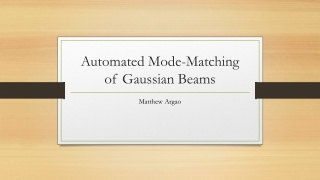Download PresentationAutomated Mode-Matching of Gaussian Beams

# Automated Mode-Matching of Gaussian Beams

Download Presentation## Automated Mode-Matching of Gaussian Beams

- - - - - - - - - - - - - - - - - - - - - - - - - - - E N D - - - - - - - - - - - - - - - - - - - - - - - - - - -
##### Presentation Transcript

1. Automated Mode-Matching of Gaussian Beams Matthew Argao

2. How do we describe a Gaussian Beam? • Main Parameters: • Waist • Radius • Complex Beam Parameter Introduction - Gaussian Beams

3. Introduction - Gaussian Beams

4. Introduction - Gaussian Beams

5. Introduction – Complex Beam Parameter How do we manipulate Gaussian beams?

6. Introduction – Complex Beam Parameter How do we manipulate Gaussian beams?

7. Introduction – Ray Matrix Analysis

8. Introduction – Ray Matrix Analysis Input Output

9. Introduction – Ray Matrix Analysis

10. Introduction – Ray Matrix Analysis • Same concept, applied to complex beam parameter

11. Focusing Gaussian Beams s = object distance s’ = image distance f = focal length of lens

12. Focusing Gaussian Beams s = initial waist distance s’ = final waist distance f = focal length of lens s s’ Self, Sidney A. "Focusing of Spherical Gaussian Beams." APPLIED OPTICS 22.5 (1983): 658-61.

13. Mode-Matching • What is it? • Why? • How? • Matrix Laboratory (MATLAB)

14. Mode-Matching • Accuracy of automated solution • Physically possible solutions • Extra considerations: • Stability of solution • Collimated region between last lens and second to last lens

15. Mode-Matching – Pre-defined Constants • λ, wavelength of beam • Ltot, total length of optical system • r0, initial radius of curvature • w0, initial waist size • x0, initial position of beam • wf, desired final waist • rf, desired final radius of curvature • lens_width, physical size of lenses • Additionally, toggle-able aesthetics for visualization and associated graphs

16. Mode-Matching – Choosing Lenses • Determine what lenses of available • Array (lens_set) • Permute all possible combinations • Array (lens_permutations)

17. Mode-Matching – Initial Placement • Generate positions of lenses • Partially random • 3rd lens is placed at a distance, f (focal length), from end of optical system • 1st and 2nd lenses placed at random between x0 and position of 3rd lens • Check distance between lens • Runs n_shuffles times for each permutation lens_width = .03 Note: in meters

18. Mode-Matching – Fitness • Two stages • Preliminary check • Deep Optimization • Fminsearch utilized • Two methods • Based on Professor Mikhailov’s original code • Based on Sydney A. Self’s Gaussian beam focusing paper

19. Mode-Matching – Fitness • For both methods two factors are constant: • Within Ltot • Difference between 1st lens and initial point • Difference between last lens and final point • Lenses do not overlap • Difference between positions of lenses themselves

20. Mode-Matching – Sydney Self Based • Self’s equations allow for us to calculate the resultant image and position of the image from a given input Gaussian beam as it passes through a thin lens. • Output of this lens becomes input of next lens • Collimation code • Lenses chosen such that resultant image from 2nd lens is >> distance between 2nd and 3rd lens to ensure collimation.

21. Mode-Matching – Mikhailov Based • Create linearly spaced array within optical system • q is calculated via Ray Matrix Analysis and evaluated at each point • Compare q values of forward and backward propagation • Collimated region also desireable • To achieve this, standard deviation of waist between 2nd and 3rd lens is taken and kept to a minimum

22. Mode-Matching – Examples Poor solution

23. Mode-Matching – Examples Good solution

24. Mode-Matching – Sorting Solutions • Solutions are sorted from lowest to highest “energy” • Problem of sensitivity/threshold • User set n_truncate • value determines how many decimal places to consider to determine solution similarity

25. Mode-Matching – Determining Unique Solutions Now are the same solution Post-Truncation Solution Array Final Solution Array

26. Solution Visualization

27. Solution Visualization

28. Stability • Perturb positions of lenses and recalculate fitness • Based on the user set amount (n_hist), the program will perturb it n_hist amount of times which is then stored in a histogram array. • Area under histogram is calculated via trapz function (trapezoidal numerical integration)

29. Stability

30. Future Directions & Considerations • Continued development and tweaking of Self Based fitness check • Ray matrix analysis method vs. Analytical method • Introduction of different optical elements • Varying indices of refraction • Mirrors etc. • GUI

31. References • Alda, Javier. "Laser and Gaussian Beam Propagation and Transformation." Encyclopedia of Optical Engineering (2003): 999-1013. • Self, Sidney A. "Focusing of Spherical Gaussian Beams." APPLIED OPTICS 22.5 (1983): 658-61.